# MCAT Physical : Henderson-Hasselbalch Equation

## Example Questions

### Example Question #971 : Mcat Physical Sciences

A solution of acetic acid (pK= 4.75) has a pH of 6.75. The ratio of acid to conjugate base is __________.

100 CH3COOH to 1 CH3COO

CH3COOH to 100 CH3COO

1 CH3COO to 100 CH3COOH

0.01 CH3COOH to 100 CH3COO

100 CH3COO to 1 CH3COOH

CH3COOH to 100 CH3COO

Explanation:

Use the Henderson-Hasselbalch equation: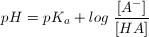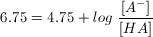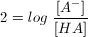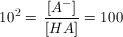We want the ratio of acid to conjugate base, which would be the reciprocal,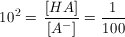### Example Question #972 : Mcat Physical Sciences

NaOH is added to a 500mL of 2M acetic acid. If the pKa value of acetic acid is approximately 4.8, what volume of 2M NaOH must be added so that the pH of the solution is 4.8?

500mL

2L

1L

250mL

250mL

Explanation:

To solve this question you need to think about the chemical reaction occurring.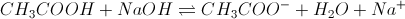We can ignore water and sodium ions for the sake of this question. The reactants exist in a 1:1 ratio, so that for every mol of NaOH we add, we lose one mol of acetic acid and gain one mol of acetate. We can determine the moles of acetic acid by using M = mol/L, which gives us mol = ML = (2M) * (0.5L) = 1mol acetic acid. If we use the Hendersen Hasselbach equation we can see that the pH equals the pKa when the concentration of conjugate base (acetate) equals the concentration of acid.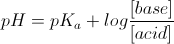If we have 1mol of acetic acid and add 0.5mol of NaOH, we will lose 0.5mol of acetic acid and gain 0.5mol of acetate. We will then be at a point where acetic acid equals acetate. This is summarized in the ICE table below. Now we know the moles of NaOH (0.5 moles) and the concentration (2M) so we can find the volume by doing M = mol/L.

L = mol/M = (0.5mol)/(2M) = 0.25L

 Acetic acid NaOH Acetate I 1 mol 0.5 mol 0 mol C -0.5 mol -0.5 mol +0.5 mol E 0.5 mol 0 mol 0.5 mol

### Example Question #1 : Henderson Hasselbalch Equation

The Ka for HCN is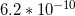.

If there is a solution of 2M HCN, what concentration of NaCN is needed in order for the pH to be 9.2?

3M

No NaCN needs to be added

2M

1M

2M

Explanation:

To answer this question, we need to be able to compare the concentrations of acid and conjugate base in the solution with the pH. The Henderson-Hasselbach equation is used to compare these values, and is written as: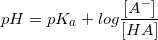. In this question, this equation can be written with the given values of Ka and pH.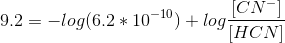Since we know the Ka of HCN, we can derive the pKa, which turns out to be 9.2.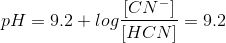As a result, we want to see to it that the amount of conjugate base is equal to the concentration of acid, so that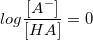. Because log(1) = 0, we want to see to it that the concentrations of the acid and the conjugate base are equal to one another. We know from the question that [HCN] = 2M.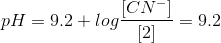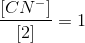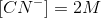As a result, a concentration of 2M NaCN will allow the pH of the solution to be 9.2.

### Example Question #4 : Henderson Hasselbalch Equation

You need to produce a buffer with pH of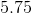. You have a solution with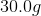of acetic acid (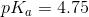). How many moles of sodium acetate must you add to achieve the desired pH?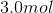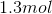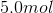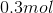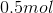Explanation:

Use the Henderson-Hasselbalch equation:Assuming that these ions occupy the same volume,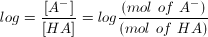We know we have 30g of acetic acid, which is equal to 0.5mol (you should memorize the formula for acetic acid).

Plugging in our values gives us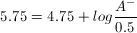Solving for A gives us 5mol.

### Example Question #5 : Henderson Hasselbalch Equation

A solution of hydrofluoric acid has a concentration of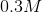.

The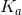for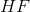is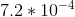.

If sodium hydroxide is slowly added to this solution, what will the pH be at the half equivalence point?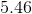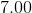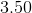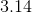Explanation:

If we use the Henderson-Hasselbalch equation, we do not need to worry about using the molar amounts of both the acid and the base. At the half equivalence point, the conjugate base concentration is equal to that of the weak acid. This means that the equation can be simplified.

Henderson-Hasselbalch equation: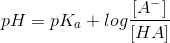Simplified equation for half equivalence point: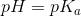Because we know the acid dissociation constant for hydrofluoric acid, the pH is calculated as: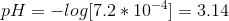### Example Question #1 : Henderson Hasselbalch Equation

Calculate the concentration of hydrogen ions in the following acetic acid solution.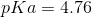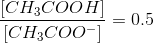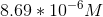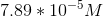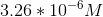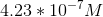Explanation:

To answer this question you need to use the Henderson-Hasselbalch equation: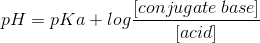The ratio given in the question is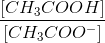, or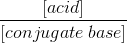.

To use the correct ratio for the Henderson-Hasselbalch equation, we need to convert this ratio to its reciprocal: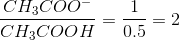Plugging the given values into the equation gives us: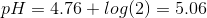The question is asking for the concentration of hydrogen ions. To solve for this we have to use the definition of pH.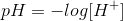Solving for the concentration of hydrogen ions gives us: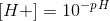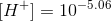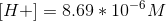### Example Question #7 : Henderson Hasselbalch Equation

Which of the following is true regarding the Henderson-Hasselbalch equation?

I. The pH of the solution is always greater than the pKa of the solution

II. As the ratio of conjugate base to acid increases, the pH increases

III. The hydrogen ion concentration can never equal the acid dissociation constant

II and III

I and II

I only

II only

II only

Explanation:

The Henderson-Hasselbalch equation is a tool that allows us to calculate the pH of an acid solution using the pKa of the acid and the relative concentrations of the acid and its conjugate base. It is defined as: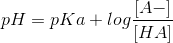By looking at the equation we can determine that if the ratio inside the logarithm is greater than 1, then the pH of the solution will be greater than the pKa; however, if the ratio is less than 1 (meaning, if the concentration of the acid is greater than the concentration of conjugate base), then the pH will be less than the pKa. Statement I is false.

Increasing the ratio of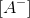to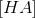will increase the logarithm, and subsequently the pH of the solution. This makes sense because you will have more conjugate base than acid, thereby making the solution more alkaline and increasing the pH. Statement II is true.

pH and pKa are defined as follows: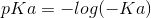If we have the same concentration of hydrogen ions as the acid dissociation constant (), then the pH will equal the pKa. According to the Henderson-Hasselbalch equation, the pH equals the pKa if the concentration of the conjugate base equals the concentration of acid; therefore, it is possible for the hydrogen ion concentration to equal the acid dissociation constant. Statement III is false.

### Example Question #1 : Henderson Hasselbalch Equation

Increasing the volume of an acid solution __________ the pH of the solution and __________ the pKa of the acid.

will increase . . . will decrease

will not alter . . . will decrease

will not alter . . . will not alter

will increase . . . will not alter

will increase . . . will not alter

Explanation:

The definition of pH is as follows:The pH of a solution heavily depends on the concentration of hydrogen ions. Recall that the concentration is in molarity (M), which is defined as: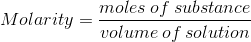Increasing the volume of the solution will decrease the concentration (molarity) of the hydrogen ions which will, subsequently, increase the pH; therefore, increasing volume will increase the pH.

Recall that pKa of an acid can never be altered. pKa is a reflection of the strength of the acid, which stays constant under all circumstances.

### Example Question #1 : Henderson Hasselbalch Equation

A researcher prepares two solutions. Solution A contains an unknown acid, HA, and solution B contains an unknown acid, HB. The researcher performs several tests and collects the following data.

1. Both solutions contain weak acids

2.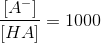3.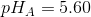4.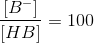5.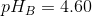What can you conclude about these two solutions?

The acids, HA and HB, are identical

The hydrogen ion concentration of solution A is greater than that of solution B

Acid HB is more acidic than acid HA

The acid dissociation constant for HA is greater than that of HB

The acids, HA and HB, are identical

Explanation:

The Henderson-Hasselbalch equation states that: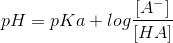The question gives us information regarding the ratio of conjugate base to acid AND the pH for each acidic solution. Using this information, we can solve for the pKa values of both solutions.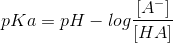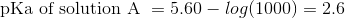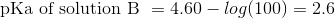The pKa values of both solutions are the same. This means that both solution contains the same acid; therefore, the identity of HA is the same as the identity of HB.

The hydrogen ion concentration of solution A is lower than that of solution B because the pH of solution A is greater. Acidity, or strength, of an acid is determined by the pKa. Since we have the same pKa for both acids, HA and HB will have the same acidity. Acid dissociation constant, Ka, is defined as: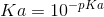Acid dissociation constant only depends on the pKa; therefore, the Ka for both acids is the same.